# (DeepLearning MOOC) Lesson 3: Convolutional Neural Networks

statistical invariance → weight sharing
e.g. image colors, translation invariance...

## convnet

is NNs that share their weights across space.

convolution: slide a small patch of NN over the image to produce a new "image"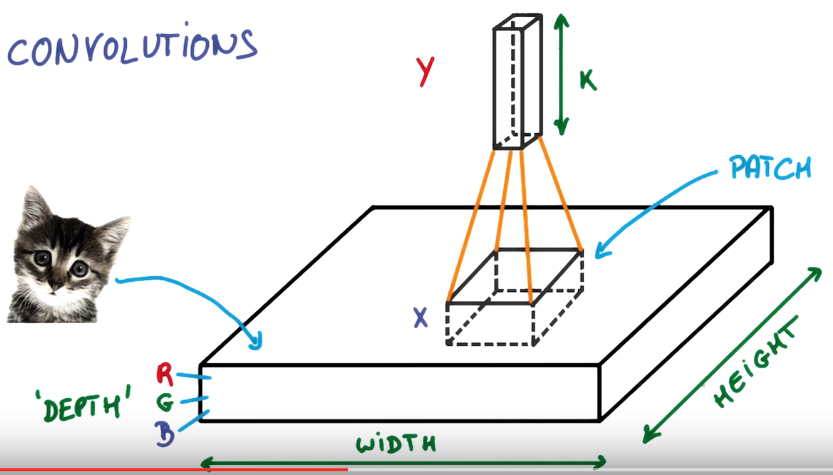convnet forms a pyramid, each "stack of pincake" get larger depth and smaller area.## convolutional lingo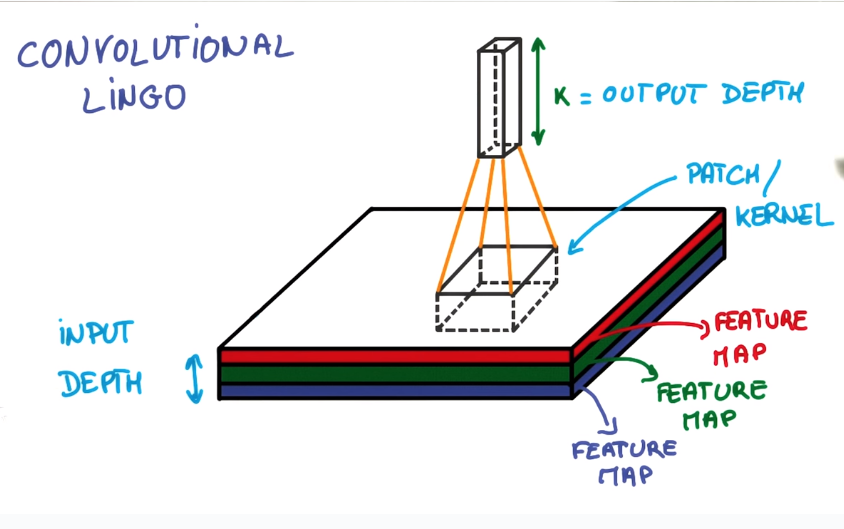def. patch (kernel)
small NN that slides over the image.

def. depth
number of pincakes in stack.

def. feature map
each "pincake" in stack.

def. stride
nb of pixels that you shift each time you move your filter.
e.g. stride=1 → output almost the same size as the input; stride=2 → output about half size

the way you treat the edge of image.

• valid padding: don't go pass the edge
• same padding: go off the image and pad with 0s (output size=input size)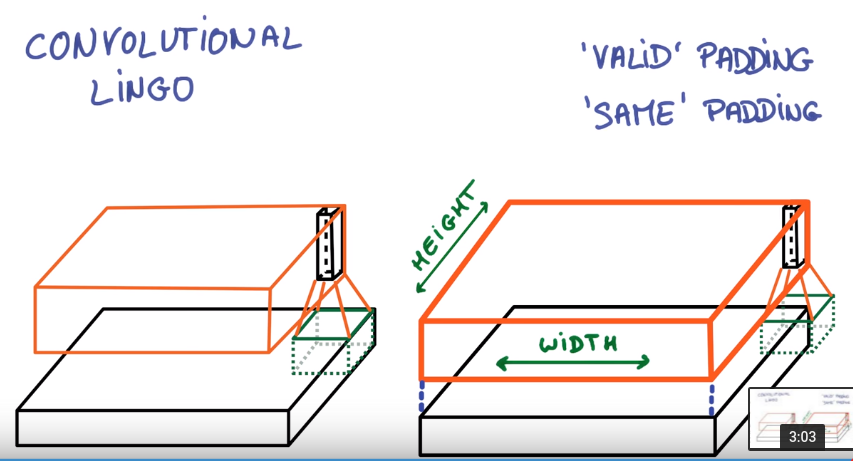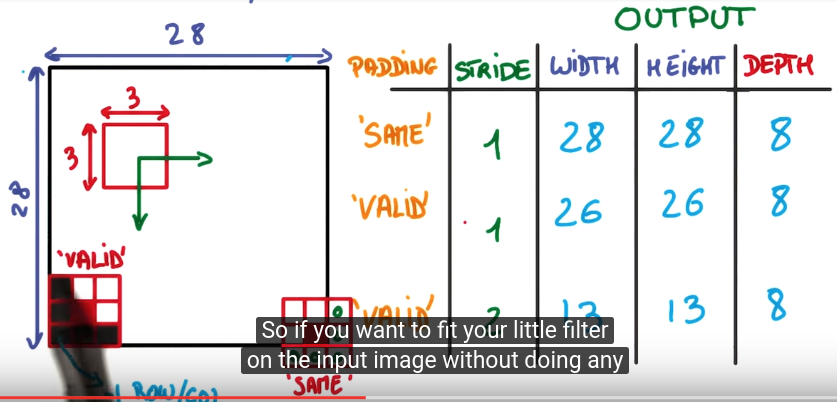once got "deep and narrow" representation by convolution, connect to a normal (regular) fully-conncected NN.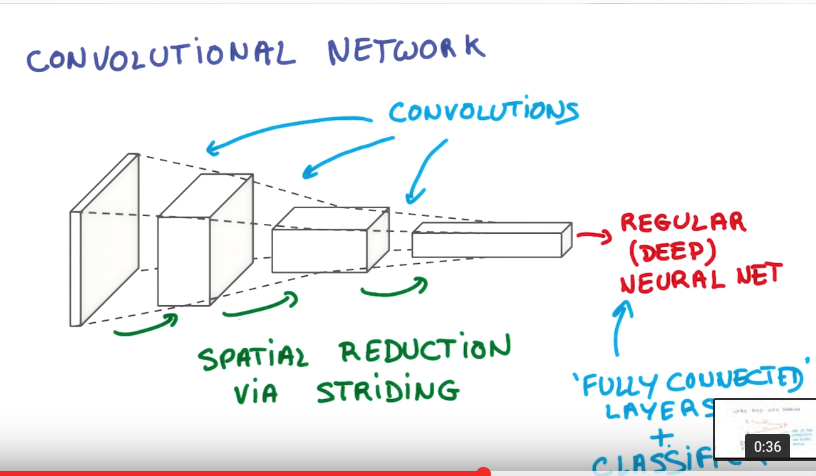## pooling

better way to reduce the spatial extend (i.e. size) of the feature map.
simple convnet: use large stride to reduce the feature map size. ⇒ aggressive
pooling: use small stride (ex. stride=1), then take convolutions in neighbourhood and combine them.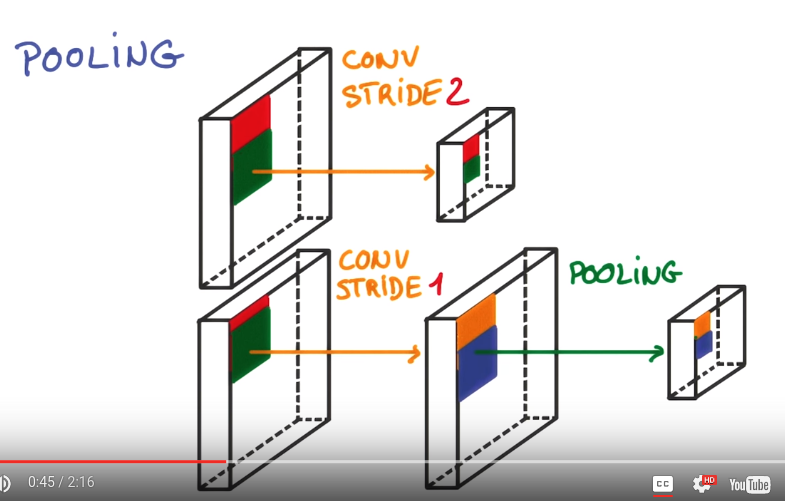max pooling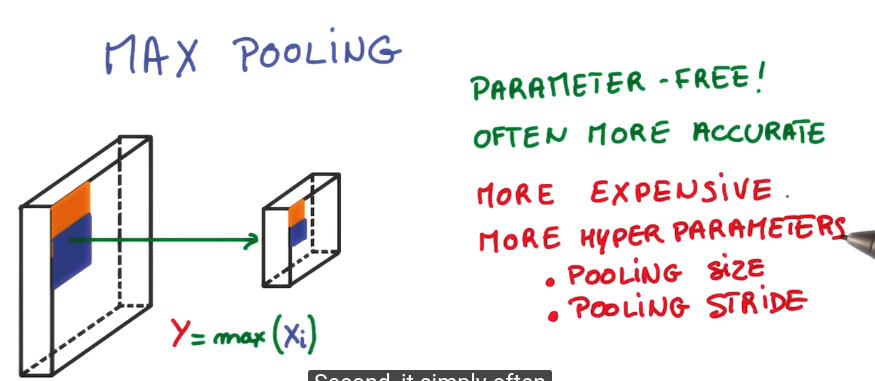average pooling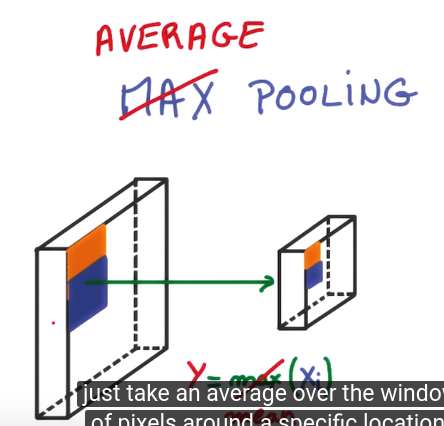## 1x1 convolution

classic convolution = linear classifier over a small patch of image
add a 1x1 convolution in the middle ⇒ a mini-dnn over the patch.
cheap: not convolution, just matrix multiplication.## inception module

between each layers, just do both pooling and 1x1 conv, and 3x3 and 5x5.. conv, and concatenate them together.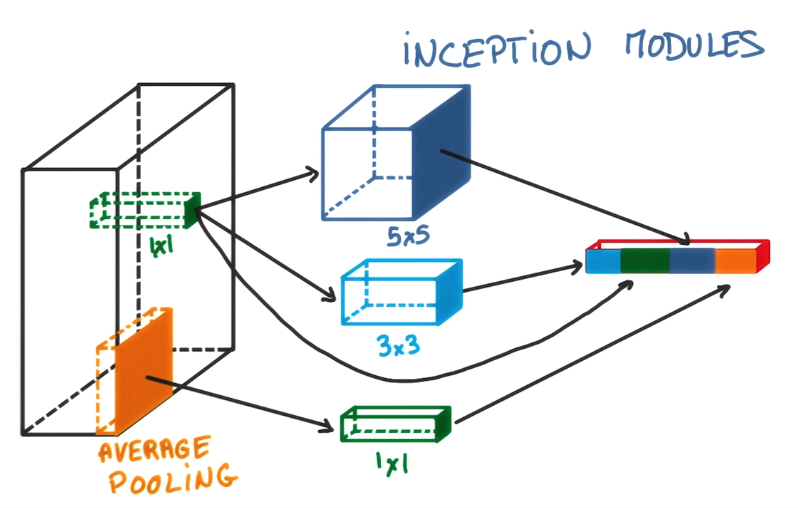benefit: total number of parameters is small, yet performance better.